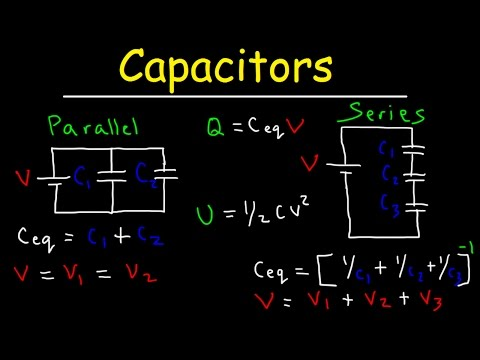Capacitors in Series and Parallel Explained

# Capacitors in Series and Parallel ExplainedCapacitors in Series and Parallel Explained

This physics video tutorial explains how to solve series and parallel capacitor circuit problems such as calculating the electric charge, voltage, and potential energy stored across each capacitor in the DC circuit network.  This video contains a few examples and practice problems with the equations / formulas and calculations needed to answer questions related to this topic.
Related Topics: